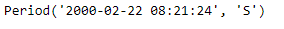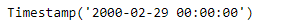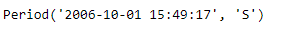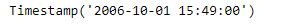Open in App
Not now

# Python | Pandas Period.to_timestamp

• Last Updated : 06 Jan, 2019

Python is a great language for doing data analysis, primarily because of the fantastic ecosystem of data-centric python packages. Pandas is one of those packages and makes importing and analyzing data much easier.

Pandas` Period.to_timestamp()` function return the Timestamp representation of the Period at the target frequency at the specified end (how) of the Period.

Syntax : Period.to_timestamp()

Parameters :
freq : Target frequency. Default is ‘D’ if self.freq is week or longer and ‘S’ otherwise
how : ‘S’, ‘E’. Can be aliased as case insensitive ‘Start’, ‘Finish’, ‘Begin’, ‘End’

Return : Timestamp

Example #1: Use `Period.to_timestamp()` function to return the given period object as a Timestamp object in the specified frequency.

 `# importing pandas as pd``import` `pandas as pd`` ` `# Create the Period object``prd ``=` `pd.Period(freq ``=``'S'``, year ``=` `2000``, month ``=` `2``, day ``=` `22``,``                         ``hour ``=` `8``, minute ``=` `21``, second ``=` `24``)`` ` `# Print the Period object``print``(prd)`

Output :Now we will use the `Period.to_timestamp()` function to return the given period object as a timestamp object.

 `# return as a timestamp in the specified frequency.``# 'M' represents monthly frequency``prd.to_timestamp(freq ``=``'M'``)`

Output :As we can see in the output, the `Period.to_timestamp()` function has returned the given period object as a timestamp in the specified frequency.

Example #2: Use `Period.to_timestamp()` function to return the given period object as a Timestamp object in the specified frequency.

 `# importing pandas as pd``import` `pandas as pd`` ` `# Create the Period object``prd ``=` `pd.Period(freq ``=``'S'``, year ``=` `2006``, month ``=` `10``,``               ``hour ``=` `15``, minute ``=` `49``, second ``=` `17``)`` ` `# Print the object``print``(prd)`

Output :Now we will use the `Period.to_timestamp()` function to return the given period object as a timestamp object.

 `# return as a timestamp in the specified frequency.``# 'T' represents minutely frequency``prd.to_timestamp(freq ``=``'T'``)`

Output :As we can see in the output, the `Period.to_timestamp()` function has returned the given period object as a timestamp in the specified frequency.

My Personal Notes arrow_drop_up